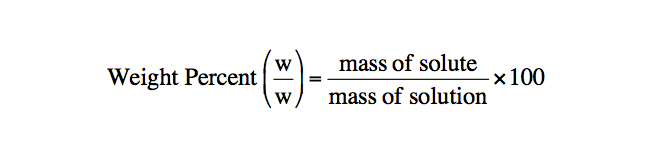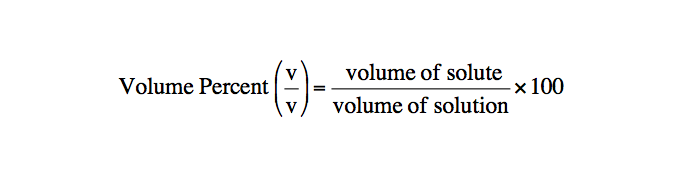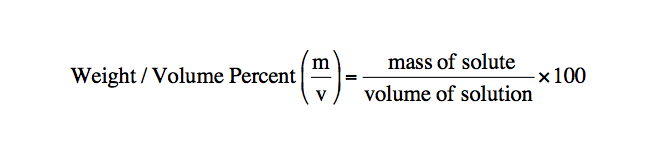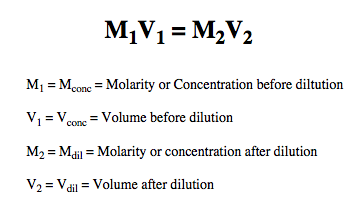Ch.1 - Chemical MeasurementsWorksheetSee all chapters
 Ch.1 - Chemical Measurements 2hrs & 10mins 0% complete Worksheet Ch.2 - Tools of the Trade 1hr & 26mins 0% complete Worksheet Ch.3 - Experimental Error 1hr & 51mins 0% complete Worksheet Ch.4 + 5 - Statistics, Quality Assurance and Calibration Methods 2hrs & 1min 0% complete Worksheet Ch.6 - Chemical Equilibrium 3hrs & 43mins 0% complete Worksheet Ch.7 - Activity and the Systematic Treatment of Equilibrium 1hr & 1min 0% complete Worksheet Ch.8 - Monoprotic Acid-Base Equilibria 1hr & 52mins 0% complete Worksheet Ch.9 - Polyprotic Acid-Base Equilibria 2hrs & 16mins 0% complete Worksheet Ch.10 - Acid-Base Titrations 2hrs & 22mins 0% complete Worksheet Ch.11 - EDTA Titrations 1hr & 37mins 0% complete Worksheet Ch.12 - Advanced Topics in Equilibrium 1hr & 18mins 0% complete Worksheet Ch.13 - Fundamentals of Electrochemistry 2hrs & 27mins 0% complete Worksheet Ch.14 - Electrodes and Potentiometry 55mins 0% complete Worksheet Ch.15 - Redox Titrations 1hr & 14mins 0% complete Worksheet Ch.16 - Electroanalytical Techniques 1hr & 23mins 0% complete Worksheet Ch.17 - Fundamentals of Spectrophotometry 55mins 0% complete Worksheet BONUS: Chemical Kinetics Not available yet

Volumetric Analysis

See all sections
Sections
SI Units
Metric Prefixes
Chemical Concentrations
Volumetric Analysis
Volumetric Titrations

The concentration of solutions can be expressed in terms beyond molarity and molality.

Percent Composition

Concept #1: The concentration of solutions can further be discussed when reviewing weight percentvolume percent, and weight/volume percent

Weight percent represents the mass composition of solute within a solution.Volume Percent represents the volume composition of solute within a solution.Weight/Volume Percent combines aspects of both weight percent and volume percent.Example #1: A solution is prepared by dissolving 18.83 g sulfuric acid, H2SO4, in enough water to make 250 ml of solution. If the density of the solution is 1.1094 g/mL, what is the weight % H2SO4 in the solution? (MW of H2SO4 is 98.086 g/mol).

Example #2: When lead levels in blood exceed 0.80 ppm (parts per million) the level is considered dangerous. 0.80 ppm means that 1 million g of blood would contain 0.80 g of Pb. Given that the density of blood is 1060.0 kg/m3, how many grams of Pb would be found in 550.00 mL of blood with a Pb level of 0.583 ppm?

Percent Composition Calculations

Example #3: A 8.13% Al2(SO4)3 solution ( MW of Al2(SO4)3 is 342.17 g/mol ) has a measured density of 1.235 g/mL. Calculate the molar concentration of sulfate ions in the solution.

Example #4: The density of a 33.8% solution of sodium acetate, NaC2H3O2, is 1.10 g/mL. A reaction requires 68.8 g NaC2H3O2. What volume of the solution do you need if you want to use a 50% excess of NaC2H3O2? (MW of NaC2H3O2 is 82.034 g/mol).

Practice: How many grams of nitric acid, HNO­3, and water are found in 53.1 g of 83.1 mass percent nitric acid?

Dilutions

Example #5: A dilution involves the addition of water to a solution in order to make it less concentrated.Example #6: How many grams of 53.1 weight % NaCl (MW of NaCl is 58.443 g/mol) should be diluted to 2.50 L to make 0.15 M NaCl?

Practice: If 920 mL of water is added to 78.0 mL of a 1.28 M HBrO4 solution what is the resulting molarity?

Dilutions Calculations

Example #7: Calculate the density of 15.9 mL of a 49.1% by weight of aqueous perchloric acid, HClO4, when it is diluted to 825 mL of 0.100 M HClO4? (MW of HClO4 is 100.461 g/mol)

Example #8: The density of 63.7 wt% NaOH is 0.915 g/mL. How many milliters of water should be diluted to 850.0 mL to create 0.425 M NaOH?